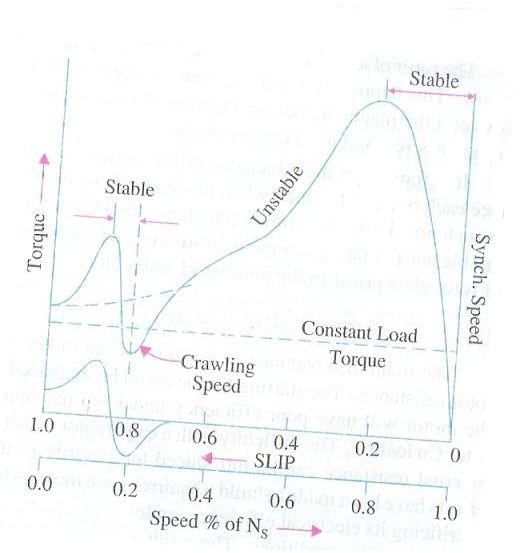# AC induction motors and the phenomenon of crawling

## Introduction

We understand what exactly is meant by the term crawling as applied to living organisms but there is a similar effect in the world of ac induction motors, so we will try and understand what exactly does the term crawling specify in context of these motors.

Before delving deeper into the concept of crawling, we must understand what exactly is meant by the term synchronous speed of a motor. We know that electrical motors turn as the rotating magnetic flux is induced to the AC current which in turn makes the rotor rotate. Ideally the speed of the rotor would be equal to the speed of flux rotation but it is not so in actual practice.

The speed at which the stator flux rotates is known as the synchronous speed, whilst the speed of actual rotation of the rotor is obviously known as actual speed, the difference between the two being known as the slip for that motor. This relation can be expressed mathematically as follows

Slip = Synchronous speed – Actual speed

Percentage Slip = {Sync speed – Actual speed}*100/Sync speed

## Crawling

Coming back to the question of crawling, it has been observed that in induction motors especially the squirrel cage type motors, there is a tendency of the motor to run stably as speeds which are nearly one-seventh of the synchronous speed and this phenomenon is known as crawling. If we think in terms of the above equation, crawling occurs when

actual speed = 1/7 * sync speed

or in other words when the percentage slip = 85.71%

So basically the definition of crawling is quite simple but why does a motor rotate at 1/7th of the sync speed or why does it crawl?

Let us take a look at the reasons behind crawling by observing the diagram shown below which shows the torque vs. % speed of the motor. The diagram is clearly marked and is fairly self descriptive with the various regions of stable and unstable rotation shown with red coloured arrows. Please correlate that diagram to what I am explaining now.We know that the winding of the motor is used to produce rotating flux which acts as a driving force to rotate the stator. Ideally this flux should be a pure sine wave but in actual practice it is not so. Rather it is a complex wave which consists not only of the fundamental frequency but also contains odd harmonics which either rotate in forward or back direction at a speed which is in proportion to the harmonic. So for example say a 7th harmonic would revolve at a speed of Ns/7 where Ns is the synchronous speed.

The main odd harmonics which effect rotor motion are the 3rd, 5th and 7th harmonic waves. Even out of these, the 3rd harmonic is normally not present in a balanced 3 phase system so only 5th and 7th come in the actual picture.

Out of these two the braking effect produced by the 5th harmonic is insignificant since they have a phase difference of -120 degrees in the three stator windings, and it revolves at Ns/5 speed in opposite direction of main harmonic.

Similarly the 7th harmonic phase difference in three stator windings is +120 degrees and there is a forward rotating field which speed Ns/7 in this case.

So the overall effect is the combination of the main harmonic and this 7th harmonic. Now if you see the diagram again you will notice that the 7th harmonic reaches its maximum positive value just before the 1/7th synchronous speed and becomes negative after that. Hence the resultant torque shows a dip as can be identified in the diagram. So if the motor is loaded at constant torque due to external mechanical engagement, it is quite possible that motor torque is lower than that applied torque. In this case the motor will not accelerate to its normal speed but continue to rotate at 1/7 speed which is exactly what crawling means.